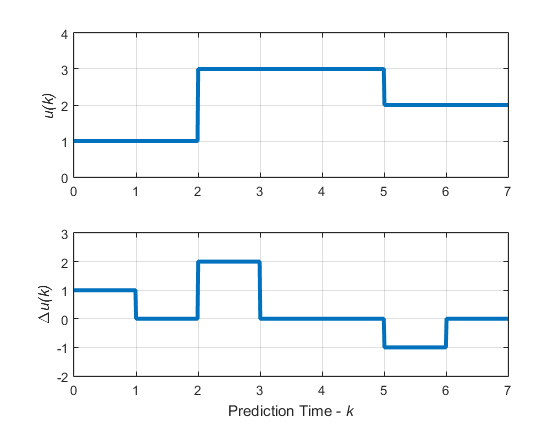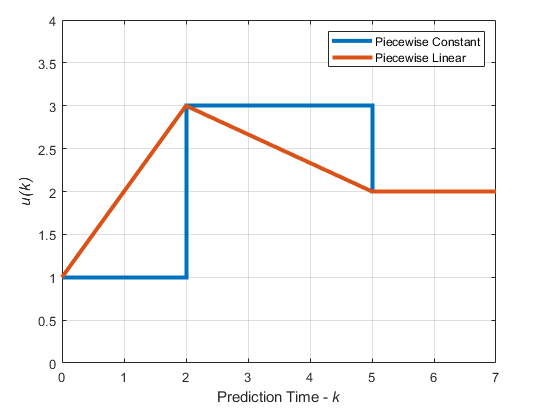Documentation

### This is machine translation

Mouseover text to see original. Click the button below to return to the English version of the page.

## Manipulated Variable Blocking

Manipulated variable blocking is an alternative to the simpler control horizon concept (see Choose Sample Time and Horizons), and it has many of the same benefits. Manipulated variable blocking:

• Provides more tuning flexibility

• Can smooth manipulated variable adjustments

• Can improve controller robustness

### Specify Blocking Interval Lengths

To use manipulated variable blocking, divide the prediction horizon into a series of blocking intervals by specifying your control horizon as a vector of block sizes, [m1, m2, …]. The sum of the block sizes must match the prediction horizon p. If you specify a vector whose sum is:

• Less than the prediction horizon, then the controller adds a blocking interval. The length of this interval is such that the sum of the interval lengths is p. For example, if p=`10` and you specify a control horizon of m=`[1 2 3]`, then the controller uses four intervals with lengths `[1 2 3 4]`.

• Greater than the prediction horizon, then the intervals are truncated until the sum of the interval lengths is equal to p. For example, if p=`10` and you specify a control horizon of `[1 2 3 6 7]`, then the controller uses four intervals with lengths `[1 2 3 4]`.

The controller computes M free moves, where M is the number of blocking intervals. The first free move applies to times k through k+m1-1, the second free move applies from time k+m1 through k+m1+m2-1, and so on. Here, k is the current control interval.

By default, the controller then holds the manipulated variable constant within each block; that is, the control moves are piecewise constant across each interval. For example, the following figure shows the optimal control moves for a control horizon of m=`[2 3 2]` and prediction horizon of p=`7`.For each block, the manipulated variable, u, is constant, that is:

• u(0) = u(1)

• u(2) = u(3) = u(4)

• u(5) = u(6)

The recommended approach to blocking is to divide the prediction horizon into 3 to 5 blocks and use one of the following blocking alternatives:

• Equal block sizes (one-fifth to one-third of the prediction horizon, p)

• Block sizes increasing. For example, with p=`20`, you can try three blocks with intervals of length 3, 7, and 10.

To test the effects of different manipulated variable blocking configurations, perform closed-loop simulation tests under the following conditions:

• No constraints

• No prediction error; that is, the controller prediction model should be identical to the plant model

To test each controller for stability and robustness issues, use the `review` function.

### Interpolate Block Moves for Nonlinear MPC

As with a linear MPC controller, when you use manipulated variable blocking, a nonlinear MPC controller uses piecewise constant blocking intervals by default. This approach is often too restrictive for optimal path planning applications. To produce a less-restrictive, better-conditioned nonlinear programming problem, you can specify piecewise linear manipulated variable blocking intervals. To do so, set the `Optimization.MVInterpolationOrder` property of your `nlmpc` controller object to `1`.

The following figure shows the optimal control moves for control horizon m=`[2 3 2]` and prediction horizon p=`7`.In the default piecewise constant case, the computed manipulated variable values of `1`, `3`, and `2` are constant over their respective blocking intervals.

In the piecewise linear case, the computed manipulated variable values are linearly interpolated for the first two blocking intervals and held constant for the final interval.

### Note

Linear interpolation of blocking moves is not supported for implicit, adaptive, or gain-scheduled MPC controllers.